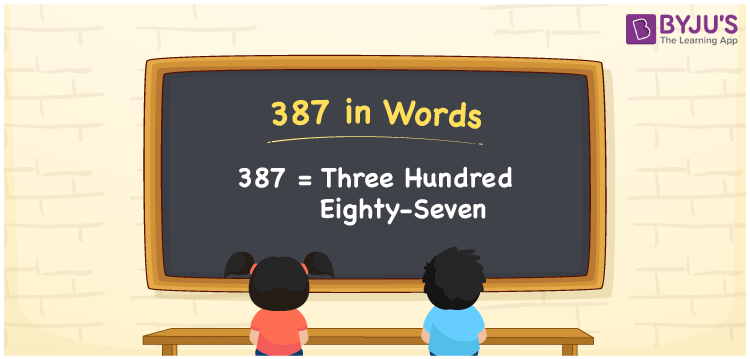# 387 in Words

387 in words is written as Three hundred eighty-seven. In both the International System of Numerals and the Indian System of Numerals, 387 is written as Three hundred eighty-seven. The number 387 is a Cardinal Number as it represents some quantity. For example, “there are 387 chairs in the room”.

 387 in Words Three hundred eighty-seven Three hundred eighty-seven in Number 387

## 387 in English Words

We write 387 in English Words using the letters of the English alphabet. Therefore, we read 387 in English as “Three hundred eighty-seven.”## How to Write 387 in Words?

To write 387 in words, we shall use the place value chart. In the place value chart, write 3 in the hundreds, 8 in the tens, and 7 in the ones, respectively. Now let us make a place value chart to write the number 387 in words.

 Hundreds Tens Ones 3 8 7

Thus, we can write the expanded form as

3 × Hundred + 8 × Ten + 7 × One

= 3 × 100 + 8 × 10 + 7 × 1

= 300 + 80 + 7

= 387

= Three hundred eighty-seven.

387 is a natural number, the successor of 386 and the predecessor of 388.

387 in words – Three hundred eighty-seven

• Is 387 an odd number? – Yes
• Is 387 an even number? – No
• Is 387 a perfect square number? – No
• Is 387 a perfect cube number? – No
• Is 387 a prime number? – No
• Is 387 a composite number? – Yes

## Frequently Asked Questions on 387 in Words

Q1

### How to write 387 in words?

387 in words is written as Three hundred eighty-seven.
Q2

### How to write 387 in the International and Indian System of Numerals?

In both, the system of numerals, 387 in words, is written as Three hundred eighty-seven.
Q3

### What is the preceding number of 387?

The number that precedes 387 is 386.